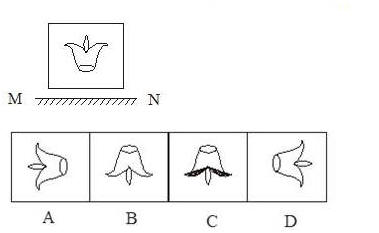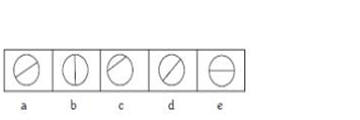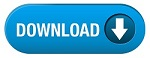IMPORTANT: RRB EXAM PORTAL is NOT associated with Railway Recruitment Board(RRB) or Indian Railways, For RRB official website visit - www.rrcb.gov.in

#a. West-east
b. South-east
c. East-north
d. North-west

## Q2. Choose the mirror image for the following figure:a. East
b. South
c. West
d. North

## Q4. What is the time between 2 o’clock and 3 o’clock at which the minute hand and the hour hand form an angle of 60° with each other?

a. 228/11 minute past 2
b. 240/11 minute past 2
c. 262/11 minute past 2
d. 250/11 minute past 2

a. Pen
b. Short
c. Socks
d. Tie

## Q6. Read the given question and decide which of the following statements is/are sufficient to answer the question?

What is the age of Sriram?
Statement:

1. Sriram is 32 years elder to Shyam.
2. Shyam is 2 years old now.

a. Both 1 and 2 are sufficient
b. Either I or 2 is sufficient
c. Only 1 is sufficient
d. Only 2 is sufficient

## Q7. A statement is given followed by two conclusions numbered I and II. Consider the given statement to be true even if it seems to be at variance with the commonly known facts and then decide which of the given conclusions logically follows from the given statement disregarding commonly known facts.

Statement: In view of further sudden increase in the international oil prices, the Government will review the present policy on fuel price

Conclusions:
I. The Government will increase the price of the fuel after the sudden increase in the international prices.
II. The Government will not increase the price of the fuel even after the sudden increase in the international oil prices.

a. Either I or II follows
b. Only conclusions II follows
c. Only conclusions I follows
d. Neither I nor II follows

a. x, x, +
b. -, x, /
c. +, /, /
d. -, /, /

a. glhb
b. hehu
c. gehu
d. heho

a. 2√73
b. √27
c. √73
d. √37

a. E
b. J
c. O
d. P

## Q12. Read the gives question and decide which of the following statement is/are sufficient /necessary to answer the question

Questions:
What is the distance between A and known C If the three towns A, B and C lie on a straight line?

Statements:
I. The distance between town B and town C is 20Km
II. The distance between town A and town B is 35 Km

a. Both I and II are necessary
b. II alone is sufficient while I alone is not sufficient
c. Neither I nor II is sufficient
d. I alone is sufficient while II alone is not sufficient.

## Q13. Consider the following statements to be true even if they seem to be at variance from commonly known facts and decide which of the conclusion logically follows, from the statements.

Statement:
No pigeon is a dove.
All doves are oriental rollers

Conclusions:
No pigeons are oriental rollers
Some oriental rollers are dove

a. Only Conclusions2 follows
b. Both conclusions 1 and 2 follow
c. Only conclusions 1 follows
d. Either conclusions 1 or 2 follows

a. Patna
b. Kohima
c. Orissa
d. Gangtok

## Q15. Consider the following question and decide which of the statement is sufficient to answer the question ]

Question: What is the measure of angle A in a right angle triangle ABC right angled at angle B?

Statement: Angle C is 20°

a. Statement 1 alone is sufficient while statement 2 alone is not sufficient
b. Each statement alone is sufficient
c. Statement 2 alone is sufficient while sufficient 1 alone is not sufficient
d. Both Statements 1 and 2 together are sufficient

## Q16. Read the given statement(s) and conclusions carefully. Assuming that the information in the statement is true, even if they appear to be in variance with commonly known facts, select which of the conclusions logically follow(s) from the statement (s) beyond reasonable doubt.

Statement : “ Sunday morning my friend cooked delicious chicken biryani, “ mentioned Sudha about her friend.

Conclusions:
I. Sudha’s friend is a good chef
II. Chicken biryani ids the only which is delicious

a. Only I follows
b. Only II follows
c. Either I or II follows
d. Neither I nor II follows

a. Gang
b. Mob
c. Items
d. Stock

a. L14N
b. L15N
c. L12N
d. N15L

## Q20. A statement is followed by two arguments. Decide which of the arguments is/are strong with respect to the statement

Statement:
Drinking tea and coffee are addictive

Arguments:
I. Yes, the caffeine in coffee or tea is a central, nervous system stimulated and it is the world’s most widely consumed addictive stimulant.
II.No, as it is widely accepted caffeine is good for health

a. Only argument II is strong
b. Neither I nor II are strong
c. Both arguments I and II are strong
d. Only argument I is strong

a. 12Km
b. 13 Km
c. 18 Km
d. 19 Km

## Q22. Take the given statement to be true and decide which of the given conclusions logically follow(s) from the statements.

Statements:
All cars are rockers. All cycles are rockers

Conclusions:
1. Some cars are cycles
2. some rockers are cycles
3. Some cycles are cars

a. None of the conclusions follows
b. Only conclusions 1 follows
c. Both conclusions 1 and 3 follow
d. Only conclusions 2 follows

a. 48F0T
b. 48F9T
c. 48F9S
d. 48G0T

## Q24. Read the given question and decide which of the following statement is/are sufficient to answer the question.

Who is standing in the middle among Y, O and P?

Statements:
1. Y is standing to the right of O.
2. P is standing to the right of Y.

a. Statements 1 and 2 together are insufficient.
b. Statement 1 alone is sufficient.
c. Statements 1 and 2 together are sufficient
d. Statement 2 alone is sufficient

a. 20
b. 0
c. 26
d. 10

a. Carbon Black
b. Coke
c. Charcoal
d. Graphite

a. NP
b. NS
c. NL
d. NK

## Q28. Choose the figure that sign different from the rest.a. b
b. e
c. c
d. d

a. Mob
b. Defect
c. Assume
d. Peace

## Q30. Consider the following statement and decide which of the conclusions logically follows from the statements.

Statements:
Cactus has thick stem and it requires less water.

Conclusions:

1. All plants with thick stem requires less water.
2. Cactus grow in places where there is less water.
3. Neither 1 nor 2 follows
4. Both 1 and 2 follows

##